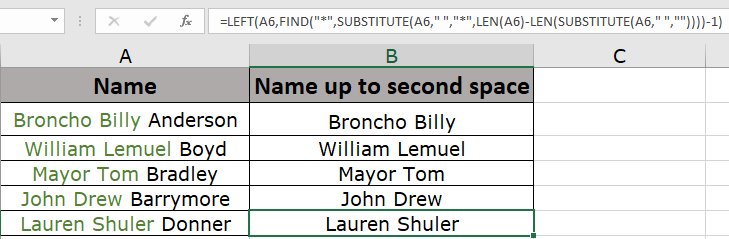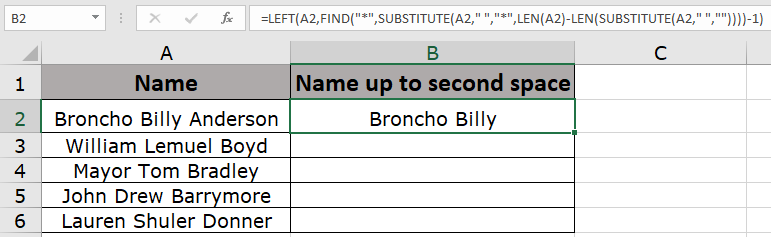# How to Return text up to the second space in Microsoft ExcelIn this article, we will learn How to Return text up to the second space in Microsoft Excel.

Extracting text up to second space (" ") character from a given string

Sometimes given a list of text strings and required to extract a part of string upto 2nd space character (" "). For example extracting the first and middle name from the full name separated by spaces. So let's understand how to solve this problem with the example explained below.

Formula

For this we will use a combination of following functions

The combination of these functions will find the second space character and replace it with any other character to which can be located easily in the manipulated string. Let's see the below given syntax with argument explanation.

Formula syntax:

 = LEFT ( string , FIND ( "*" , SUBSTITUTE ( string , " " , "*" , LEN (string) - LEN ( SUBSTITUTE (string ," " , "" ) ) ) ) - 1 )

string : given string

" " : space char

"*" : char to replace space char (must be unique for all names)

Example :

All of these might be confusing to understand. Let's understand how to use the function using an example. Here we have a list of names and we need to find the first and middle name from the full names.

Use the formula:

 = LEFT ( A2 , FIND ( "*" , SUBSTITUTE ( A2 , " " , "*" , LEN (A2) - LEN ( SUBSTITUTE (A2 ," " , "" ) ) ) ) - 1 )

Explanation

1. LEN function returns the length of the string.
2. SUBSTITUTE (A2," ", "") returns "BronchoBillyAnderson" (without spaces)
3. LEN (A2) - LEN (SUBSTITUTE (A2," ", "")) returns the difference of names with spaces and without spaces. So we get the count of spaces in the string. i.e. 2
4. The difference will be used to replace the * char to the particular space character. Returns "Broncho Billy*Anderson"
5. FIND function finds the * char and LEFT function extracts the value till * char.
6. -1 be used so that the new string doesn't contain space char. Its momentary choice to keep the space or not. Doesn't make any difference.As you can see the resulting name has only the first and middle name. Copy the formula to other cells using the shortcut Ctrl + D .As you can see the we got all names using one formula. Isn't it interesting!

 NOTE: Use the SEARCH function in place of the FIND function. Both return the same value. But FIND function is case - sensitive and doesn't support wildcards.

Here are all the observational notes using the formula in Excel
Notes :

1. Use the brackets carefully in the formula, as it might return error.
2. The * char used here. You can use any unique char to replace space characters for all strings.
3. If the name is divided by any other char (like | ) replace the given delimiter in the formula with quotes.

Hope this article about Return text up to the second space in Microsoft Excel is explanatory. Find more articles on string editing formulas and related Excel formulas here. If you liked our blogs, share it with your friends on Facebook. And also you can follow us on Twitter and Facebook. We would love to hear from you, do let us know how we can improve, complement or innovate our work and make it better for you. Write to us at info@exceltip.com.

Related Articles:

How to Use TRIM function in Excel: The TRIM function is used to trim strings and clean any trailing or leading spaces from string. This helps us in the cleaning process of data a lot.

How to use the CLEAN function in Excel: Clean function is used to clean up unprintable characters from the string. This function is mostly used with the TRIM function to clean up imported foreign data.

Replace text from end of a string starting from variable position: To replace text from the end of the string, we use the REPLACE function. The REPLACE function uses the position of text in the string to replace.

How to Check if a string contains one of many texts in Excel: To find check if a string contains any of multiple text, we use this formula. We use the SUM function to sum up all the matches and then perform a logic to check if the string contains any of the multiple strings.

Count Cells that contain specific text: A simple COUNTIF function will do the magic. To count the number of multiple cells that contain a given string we use the wildcard operator with the COUNTIF function.

Excel REPLACE vs SUBSTITUTE function: The REPLACE and SUBSTITUTE functions are the most misunderstood functions. To find and replace a given text we use the SUBSTITUTE function. Where REPLACE is used to replace a number of characters in string…

Popular Articles :

How to use the IF Function in Excel : The IF statement in Excel checks the condition and returns a specific value if the condition is TRUE or returns another specific value if FALSE.

How to use the VLOOKUP Function in Excel : This is one of the most used and popular functions of excel that is used to lookup value from different ranges and sheets.

How to Use SUMIF Function in Excel : This is another dashboard essential function. This helps you sum up values on specific conditions.

How to use the COUNTIF Function in Excel : Count values with conditions using this amazing function. You don't need to filter your data to count specific values. Countif function is essential to prepare your dashboard.

1."Far beit from me to tell a native how their own postal system works, but I *believe* you might find that the actual structure of the postal codes in the UK requires you to insert a character (space) such that the last three characters are separated from the first three or four.

This as opposed to just inserting at the fifth character.

I could very well be wrong, or out of date, but I think that some post codes are similar to:

CV4 7AL (somewhere in Coventry)

Either way, I think that if your orginal format code is in cell A1, then the following should do it:

=LEFT(A1,LEN(A1)-3)&"" ""&RIGHT(A1,3)

Apologies if I am mistaken - it is a long way down here! "

2.My problem regarding postcodes is completely the opposite. I have a list of postcodes, e.g. NH129AS but I want them to be in the format NH12 9AS (ie insert a space as the 5th character). Does anyone know how I can do this in Excel?

3."Guido's solution certainly works (although in my version of Excel, it needs commas rather than semi-colons, but that is probably a geogrpahical issue?)

However, the following is perhaps simpler, and more general:

=SUBSTITUTE(E13,"" "","""")

{Note the 'space' between the first set of quotes}

This will replace ALL the spaces in the postcode, not just the first occurance. "

4."Hi, assume E13 contains your post code

=REPLACE(E13;FIND("" "";E13);1;"""")

http://HoloGuides.com/programming/ExcelVBA"

5."Hi, I have a postcode file in Excel,

The post codes in some cells are, for example OL16 6YH. I need somehow to take the space out, So it reads OL166YH.

Anyway to do this in Excel? "

•Satish Prajapati

6."Hi Chris,
Far beit from me to tell a native how their own postal system works, but I *believe* you might find that the actual structure of the postal codes in the UK requires you to insert a character (space) such that the last three characters are separated from the first three or four.
This as opposed to just inserting at the fifth character.
I could very well be wrong, or out of date, but I think that some post codes are similar to:
CV4 7AL (somewhere in Coventry)
Either way, I think that if your orginal format code is in cell A1, then the following should do it:
=LEFT(A1,LEN(A1)-3)&"" ""&RIGHT(A1,3)
Apologies if I am mistaken - it is a long way down here!
Alan."

7.My problem regarding postcodes is completely the opposite. I have a list of postcodes, e.g. NH129AS but I want them to be in the format NH12 9AS (ie insert a space as the 5th character). Does anyone know how I can do this in Excel?

•Satish Prajapati

=CONCATENATE(LEFT(A28,4)," ",RIGHT(A28,3))

8."Hi,
Guido's solution certainly works (although in my version of Excel, it needs commas rather than semi-colons, but that is probably a geogrpahical issue?)
However, the following is perhaps simpler, and more general:
=SUBSTITUTE(E13,"" "","""")
{Note the 'space' between the first set of quotes}
This will replace ALL the spaces in the postcode, not just the first occurance.
Hope that helps,
Alan."

9.Hi, assume E13 contains your post code
=REPLACE(E13;FIND("" "";E13);1;"""")

10."Hi, I have a postcode file in Excel,
The post codes in some cells are, for example OL16 6YH. I need somehow to take the space out, So it reads OL166YH.
Anyway to do this in Excel?
Thanks!!
Matt"

Terms and Conditions of use

The applications/code on this site are distributed as is and without warranties or liability. In no event shall the owner of the copyrights, or the authors of the applications/code be liable for any loss of profit, any problems or any damage resulting from the use or evaluation of the applications/code.